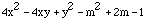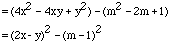Date: Sun, 21 Jun 1998 22:34:03 -0600 (CST)
Subject: mathematics A30

Name: Kristen

Level: Secondary

Question:
Solve the following using a difference of squares:

4x(squared) minus 4xy plus y(squared) minus m(squared) plus 2m minus 1

Hi Kristen
In mathematics the word "solve" applies to equations and you are being asked to find the value or values of the variable(s) that make the equation true. You don't have an equation so I am going to assume that you want to simplifyusing a difference of squares. You first need to see that you can write the expression as a difference of squares. To do this notice thatNow you have a difference of squares to factor.

Cheers
Penny

Go to Math Central